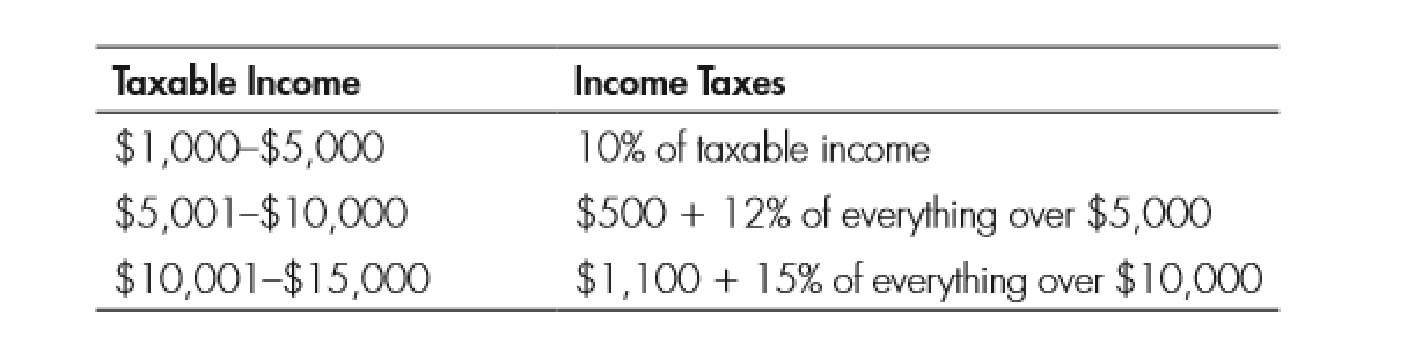Chapter 11, Problem 3WNG

Chapter
Section
Textbook Problem

Use the following table to answer questions 1-4:3. What is the marginal tax rate on the 10,001st dollar? What is the marginal tax rate on the 10,000th dollar?

To determine

Identify the marginal rate of tax.

Explanation

As per the given table, people who earn $10,000 have to pay$500 plus 12% of everything over $5,000 as tax. Since the income is$10,000, 12% of $5,000 ($10,000-$5,000) is$600($5,000×12/100). Therefore, the total amount of tax is$600 plus $500 that is$1,100.  Hence, the marginal rate for the \$10,000th dollar is 12%

Still sussing out bartleby?

Check out a sample textbook solution.

See a sample solution

The Solution to Your Study Problems

Bartleby provides explanations to thousands of textbook problems written by our experts, many with advanced degrees!

Get Started

What is publicity, and why do organizations use it?

Foundations of Business (MindTap Course List)

What is a cost object? Give some examples.

Managerial Accounting: The Cornerstone of Business Decision-Making

Suppose interest rates on Treasury bonds rose from 5% to 9% as a result of higher interest rates in Europe. Wha...

Fundamentals of Financial Management, Concise Edition (with Thomson ONE - Business School Edition, 1 term (6 months) Printed Access Card) (MindTap Course List)